# 一. 绪论

## 1.2 分类算法

(1)数据清理。其目的是消除或减少数据噪声，处理空缺值。

(2)相关性分析。由于数据集中的许多属性可能与分类任务不相关，若包含这些属性将减慢和可能误导学习过程。相关性分析的目的就是删除这些不相关或冗余的属性。

(3)数据变换。数据可以概化到较高层概念。比如，连续值属性“收入”的数值可以概化为离散值：低，中，高。又比如，标称值属性“市”可概化到高层概念“省”。此外，数据也可以规范化, ,规范化将给定属性的值按比例缩放，落入较小的区间，比如[0,1]等。

# 二. 支持向量机SVM

## 2.2 SVM的基本思想

SVM可以分为两个部分了解。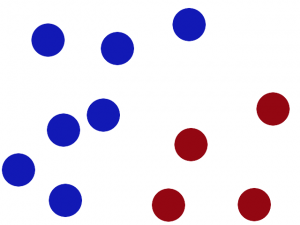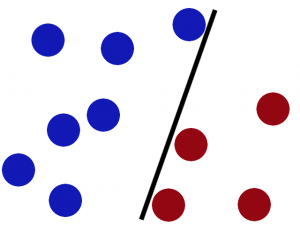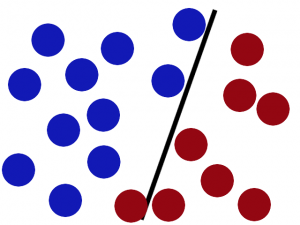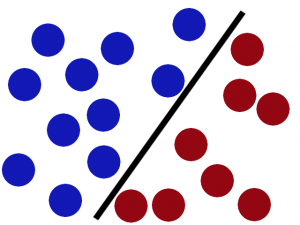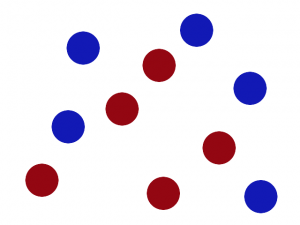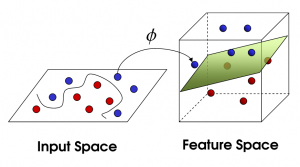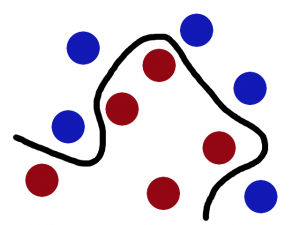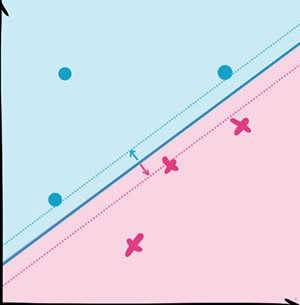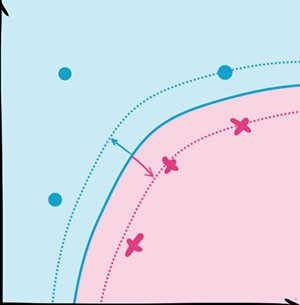## 2.3 SVM的常见模型

SVM的常见模型可以分为两种，一种是线性支持向量机，一种是非线性支持向量机。本文主要介绍线性支持向量机中的软间隔最大化模型，在介绍这种模型前，首先要了解什么是支持向量以及SVM模型的目标函数。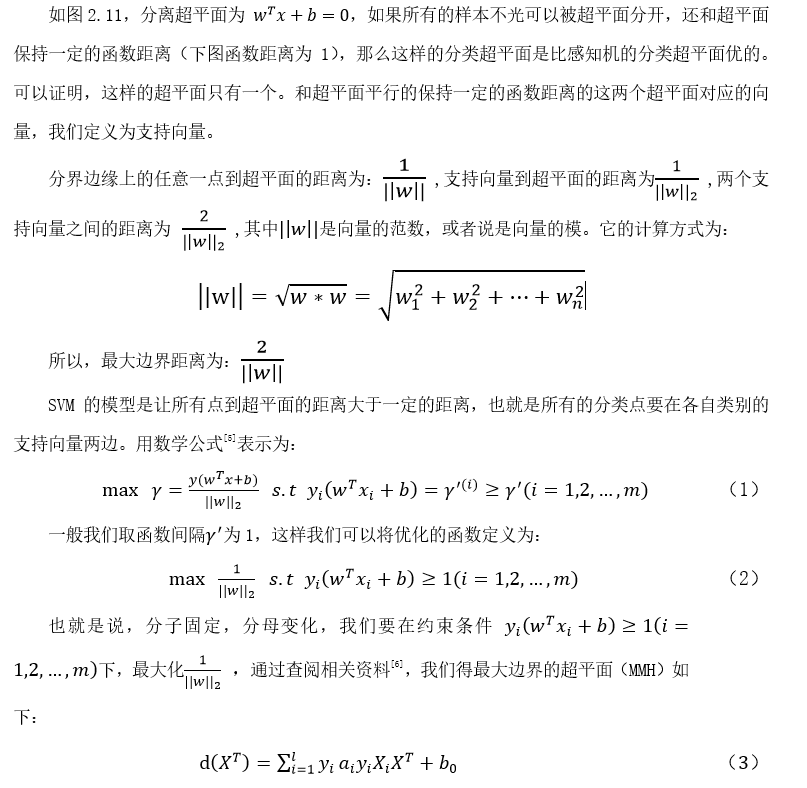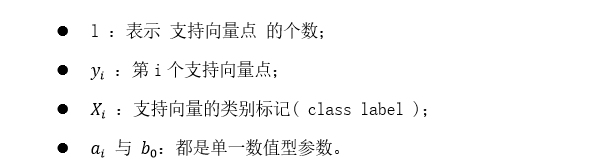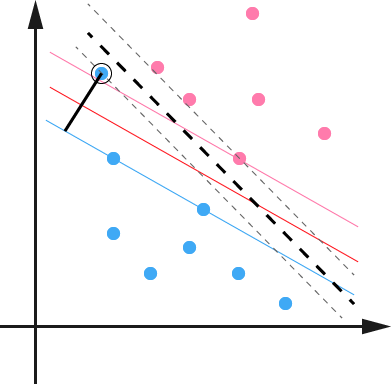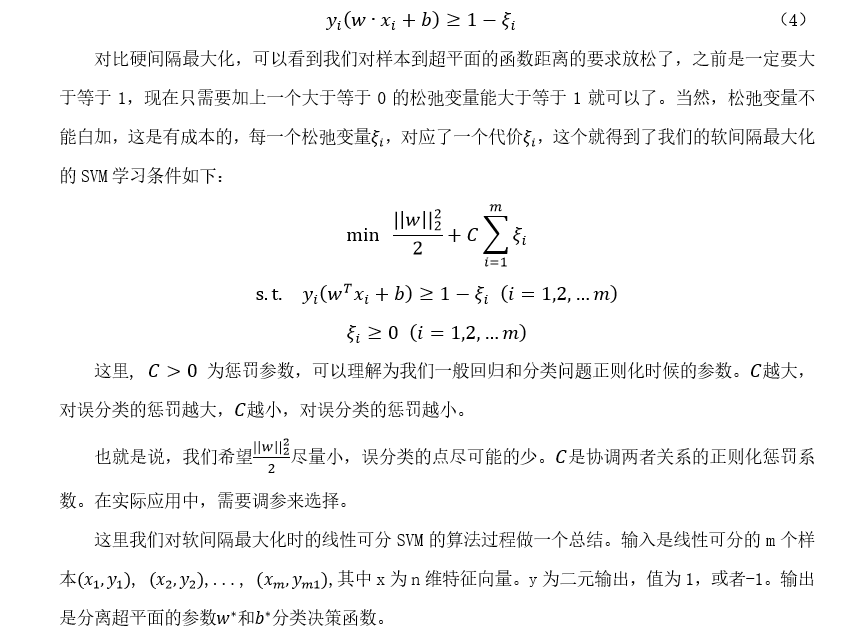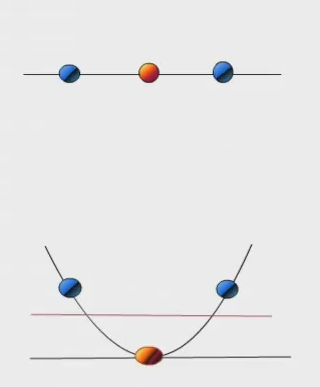# 三. 支持向量机SVM的应用

## 3.1 模型可视化展示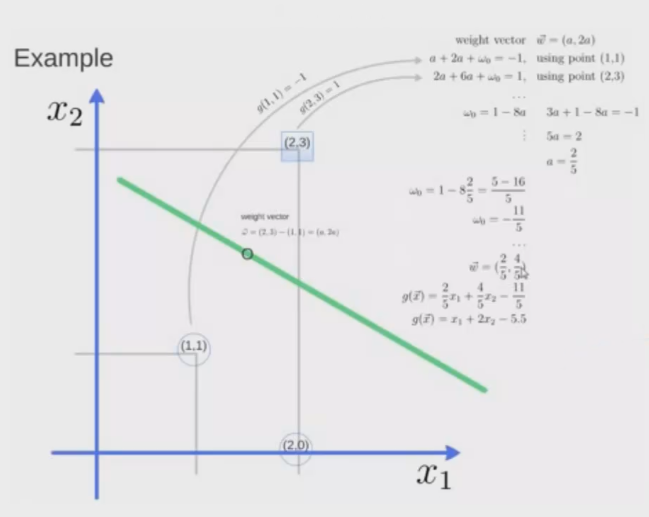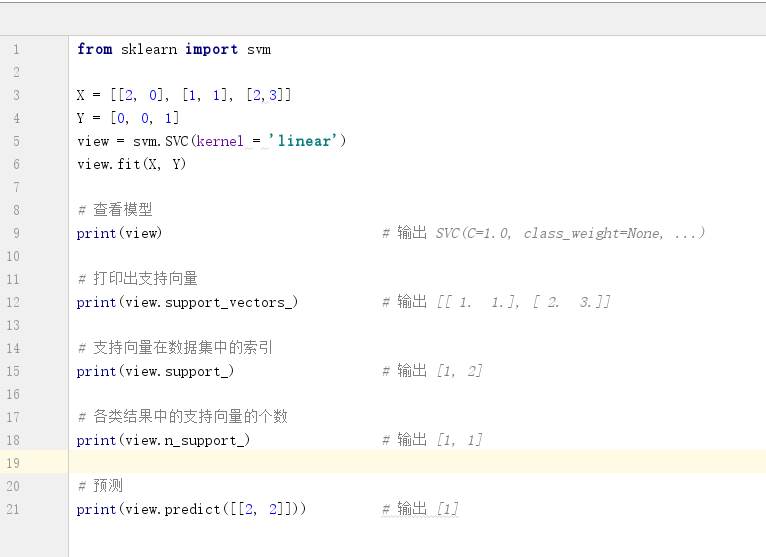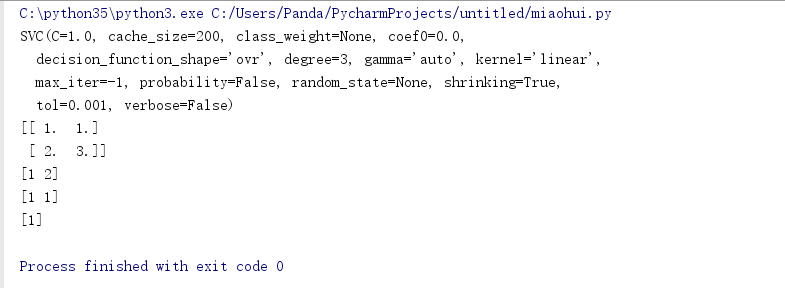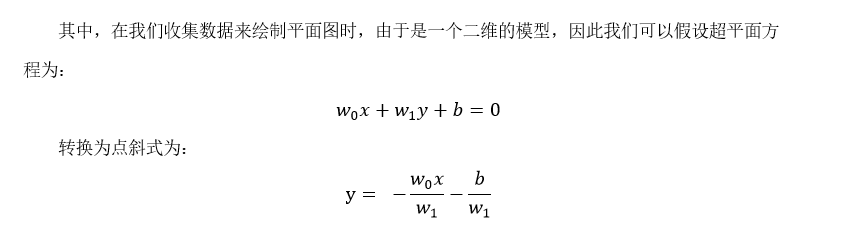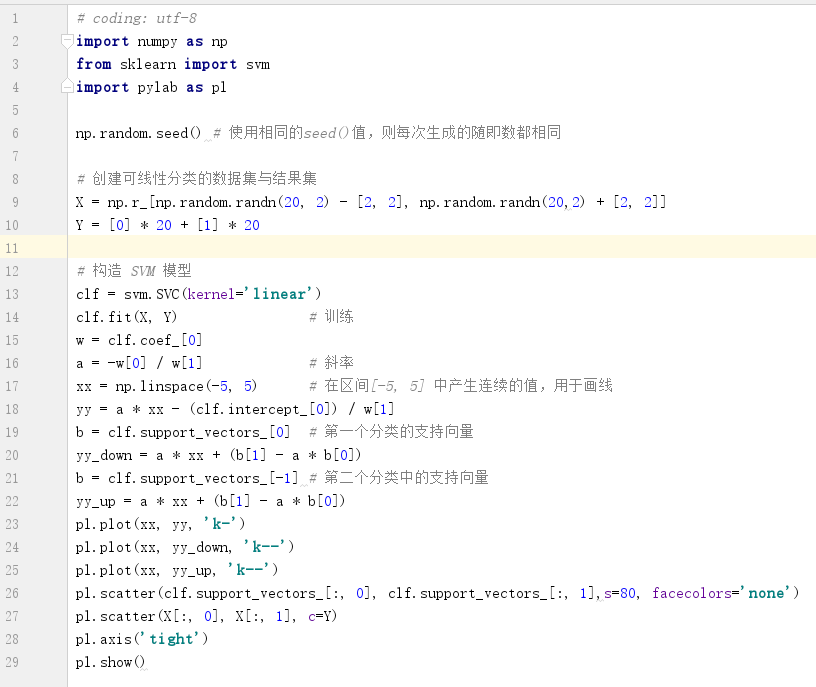``````# coding: utf-8
import numpy as np
from sklearn import svm
import pylab as pl

np.random.seed() # 使用相同的seed()值，则每次生成的随即数都相同

# 创建可线性分类的数据集与结果集
X = np.r_[np.random.randn(20, 2) - [2, 2], np.random.randn(20,2) + [2, 2]]
Y =  * 20 +  * 20

# 构造 SVM 模型
clf = svm.SVC(kernel='linear')
clf.fit(X, Y)                # 训练
w = clf.coef_
a = -w / w             # 斜率
xx = np.linspace(-5, 5)      # 在区间[-5, 5] 中产生连续的值，用于画线
yy = a * xx - (clf.intercept_) / w
b = clf.support_vectors_  # 第一个分类的支持向量
yy_down = a * xx + (b - a * b)
b = clf.support_vectors_[-1] # 第二个分类中的支持向量
yy_up = a * xx + (b - a * b)
pl.plot(xx, yy, 'k-')
pl.plot(xx, yy_down, 'k--')
pl.plot(xx, yy_up, 'k--')
pl.scatter(clf.support_vectors_[:, 0], clf.support_vectors_[:, 1],s=80, facecolors='none')
pl.scatter(X[:, 0], X[:, 1], c=Y)
pl.axis('tight')
pl.show()
``````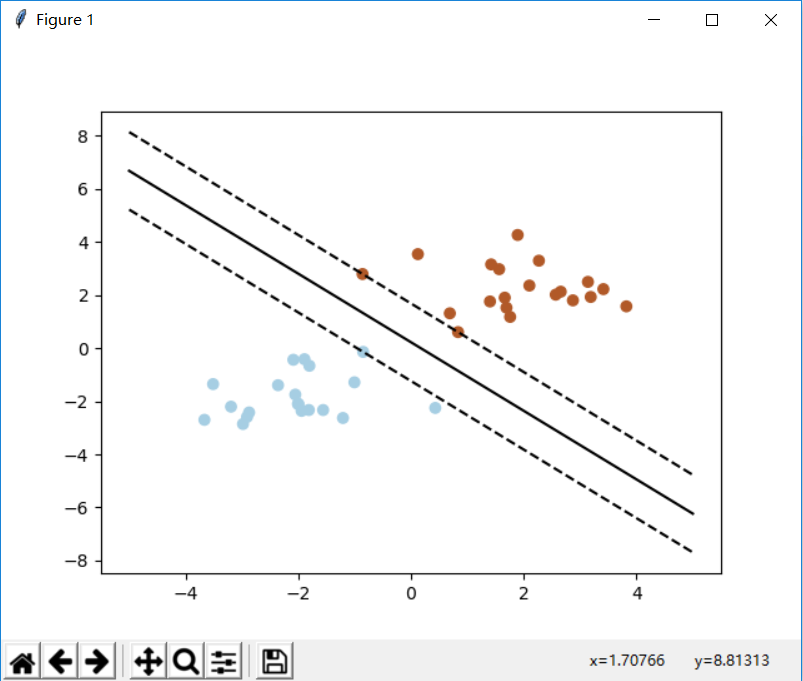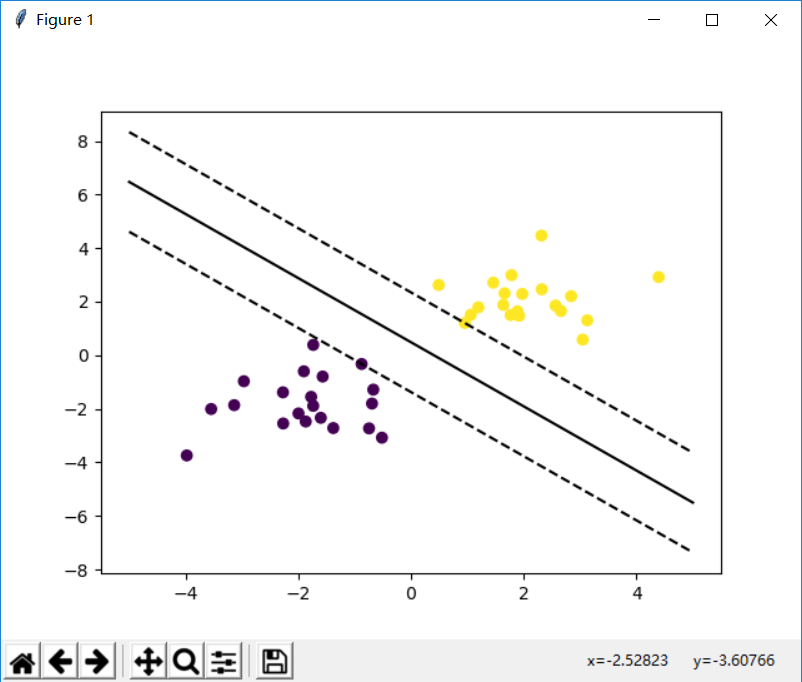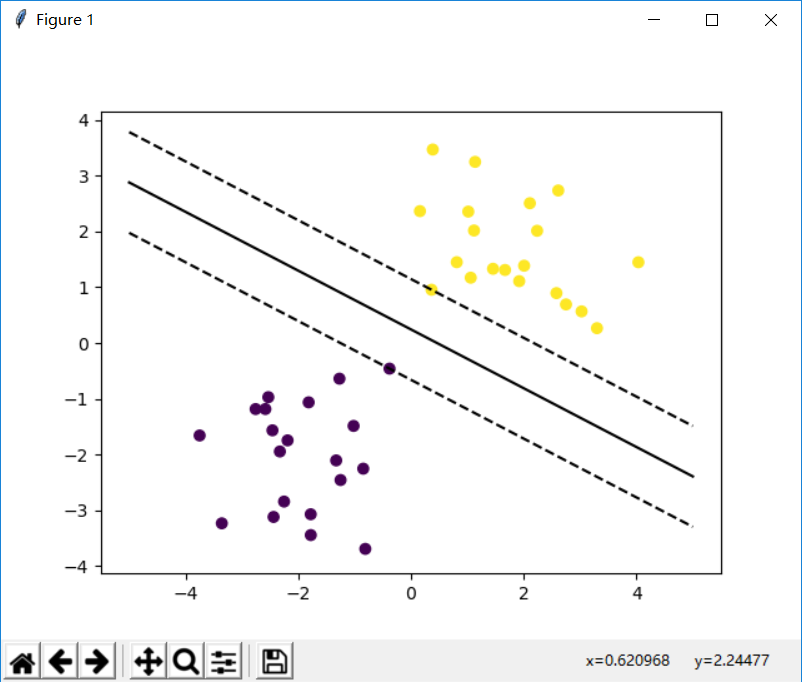## 3.2 人脸识别

（1）人脸数据集基本信息

（2）拆分训练集和测试集

X_train, X_test, Y_train, Y_test = train_test_split(X, Y,
test_size=0.25)

（3）特征降维处理

（4）提取特征点

（5）构造SVM分类器

（6）预测

（7）测试结果可视化

``````from __future__ import print_function
from time import time
import logging
import matplotlib.pyplot as plt
import cv2
from numpy import *
from sklearn.model_selection import train_test_split
from sklearn.model_selection import GridSearchCV
from sklearn.metrics import classification_report
from sklearn.metrics import confusion_matrix
from sklearn.decomposition import PCA
from sklearn.svm import SVC

#读取图像
PICTURE_PATH = "F:\\face\\"
def get_Image():
for i in range(1,41):
for j in range(1,11):
path = PICTURE_PATH + "\\s" + str(i) + "\\"+ str(j) + ".pgm"
img_gray = cv2.cvtColor(img, cv2.COLOR_BGR2GRAY)
h,w = img_gray.shape
img_col = img_gray.reshape(h*w)
all_data_set.append(img_col)
all_data_label.append(i)
return h,w

all_data_set = []
all_data_label = []
h,w = get_Image()

X = array(all_data_set)
y = array(all_data_label)
n_samples,n_features = X.shape
n_classes = len(unique(y))
target_names = []
for i in range(1,41):
names = "person" + str(i)
target_names.append(names)

print("Total dataset size:")
print("n_samples: %d" % n_samples)
print("n_features: %d" % n_features)
print("n_classes: %d" % n_classes)

# 拆分训练集和测试集
X_train, X_test, y_train, y_test = train_test_split(
X, y, test_size=0.25, random_state=42)
# 特征降维处理
n_components = 20
print("Extracting the top %d eigenfaces from %d faces"% (n_components, X_train.shape))
t0 = time()
pca = PCA(n_components=n_components, svd_solver='randomized',whiten=True).fit(X_train) #选择一种svd方式
print("done in %0.3fs" % (time() - t0))
eigenfaces = pca.components_.reshape((n_components, h, w))  #特征脸
print("Projecting the input data on the eigenfaces orthonormal basis")
t0 = time()
X_train_pca = pca.transform(X_train)  #得到训练集投影系数
X_test_pca = pca.transform(X_test)    #得到测试集投影系数
print("done in %0.3fs" % (time() - t0))
print("Fitting the classifier to the training set")
t0 = time()
param_grid = {'C': [1e3, 5e3, 1e4, 5e4, 1e5], 'gamma': [0.0001, 0.0005, 0.001, 0.005, 0.01, 0.1], }
clf = GridSearchCV(SVC(kernel='rbf', class_weight='balanced'), param_grid)
#class_weight='balanced'表示调整各类别权重，权重与该类中样本数成反比，
#防止模型过于拟合某个样本数量过大的类
clf = clf.fit(X_train_pca, y_train)
print("done in %0.3fs" % (time() - t0))
print("Best estimator found by grid search:")
print(clf.best_estimator_)

print("Predicting people's names on the test set")
t0 = time()
y_pred = clf.predict(X_test_pca)
print("done in %0.3fs" % (time() - t0))

print(classification_report(y_test, y_pred, target_names=target_names))
print(confusion_matrix(y_test, y_pred, labels=range(n_classes)))

def plot_gallery(images, titles, h, w, n_row=3, n_col=4):
plt.figure(figsize=(1.8 * n_col, 2.4 * n_row))
for i in range(n_row * n_col):
plt.subplot(n_row, n_col, i + 1)
plt.imshow(images[i].reshape((h, w)))
plt.title(titles[i], size=12)
plt.xticks(())
plt.yticks(())
def title(y_pred, y_test, target_names, i):
pred_name = target_names[y_pred[i]-1]
true_name = target_names[y_test[i]-1]
return 'Predicted: %s\nResult:      %s' % (pred_name, true_name)

prediction_titles = [title(y_pred, y_test, target_names, i)for i in range(y_pred.shape)]
eigenface_titles = ["Eigenface %d" % i for i in range(eigenfaces.shape)]
plot_gallery(X_test, prediction_titles, h, w)
plot_gallery(eigenfaces, eigenface_titles, h, w)
plt.show()
``````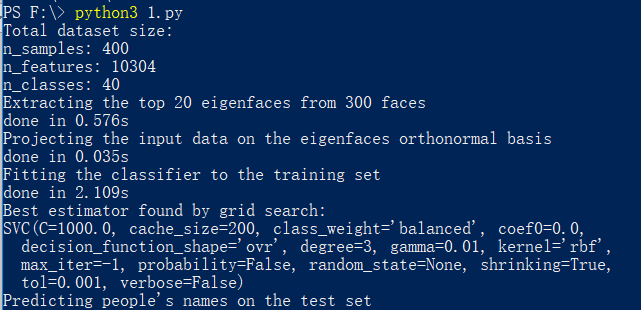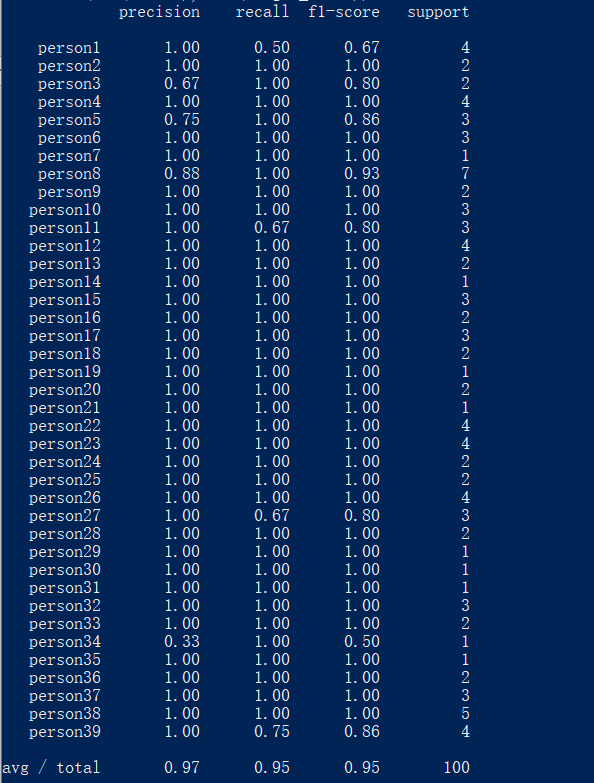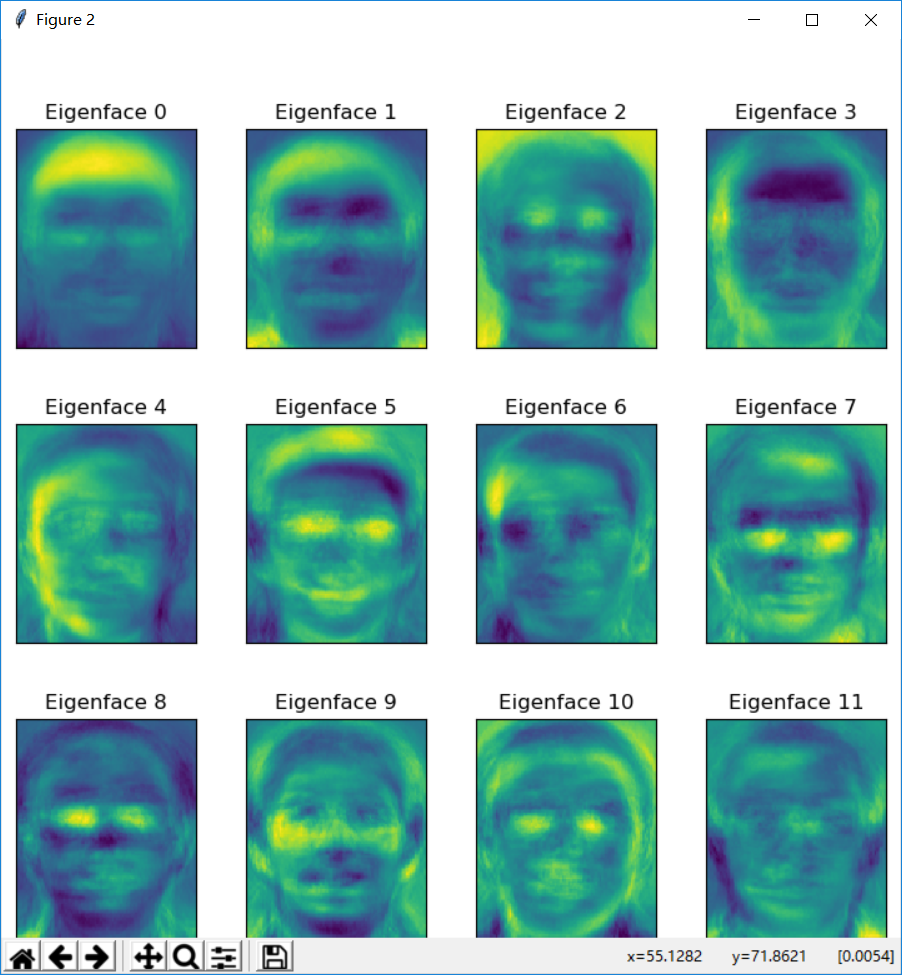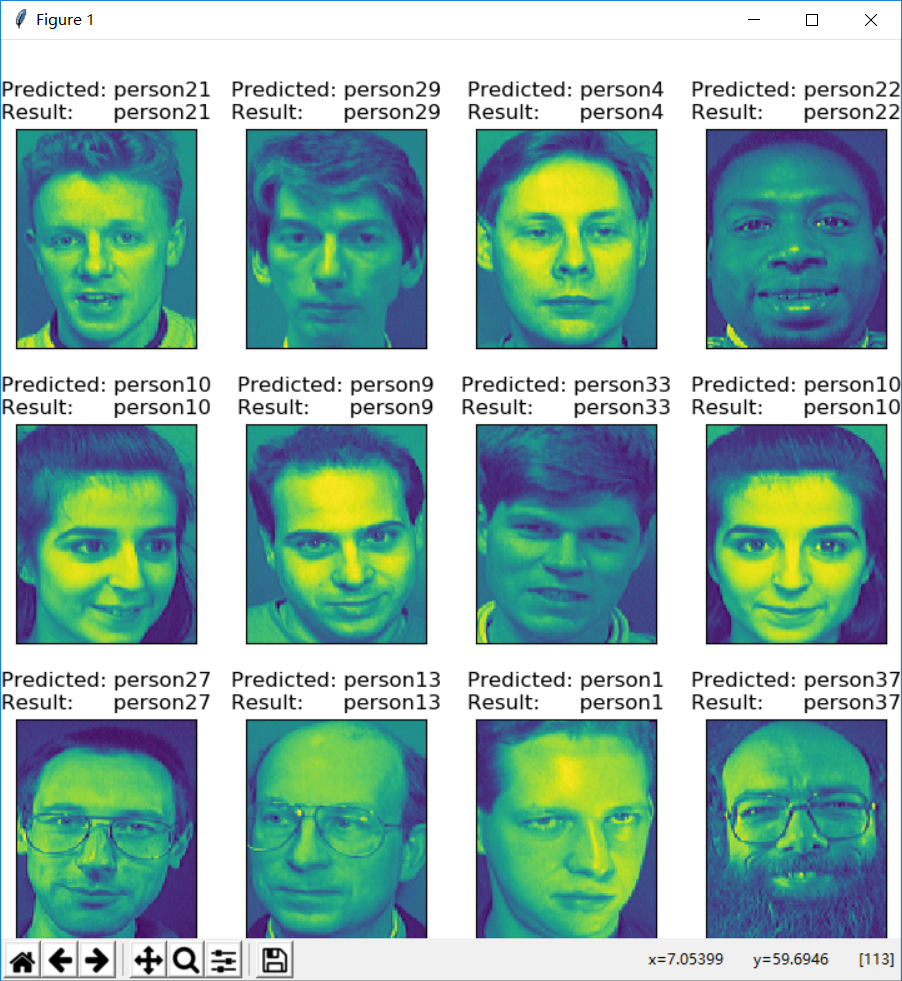SVM的主要优点包括：

# 四. 总结

## 相关下载

 刘红岩,陈剑,陈国青. 数据挖掘中的数据分类算法综述
 陶卿,曹进德,孙德敏. 基于支持向量机分类的回归方法
 罗海蛟,刘显.数据挖掘中分类算法的研究及其应用
 Iddo. Support Vector Machines explained well
 Nello Cristianini,John Shawe-Taylor. 支持向量机导论
 邓乃扬,田英杰. 数据挖掘中的新方法：支持向量机
 林轩田. 机器学习技法. MOOC
 段纪军,陈琳,王海燕,田娜.基于数据挖掘技术与支持向量机的目标识别研究
 朱凌云,曹长修.基于支持向量机的缺陷识别方法
 Support Vector Machines explained well
 支持向量机(SVM)是什么意思？
 svm 算法通俗介绍
 分类算法之支持向量机:SVM（应用篇）
 Scikit-learn实例之Pca+Svm人脸识别(AT&T数据集)

「一键投喂 软糖/蛋糕/布丁/牛奶/冰阔乐！」

(๑＞ڡ＜)☆谢谢老板~